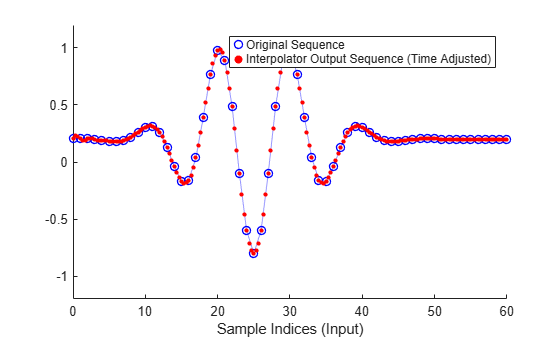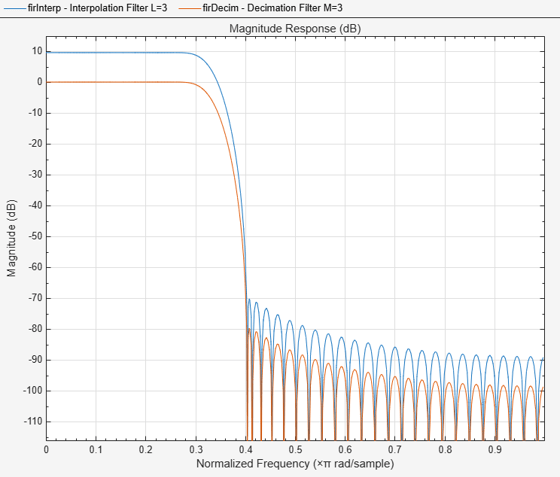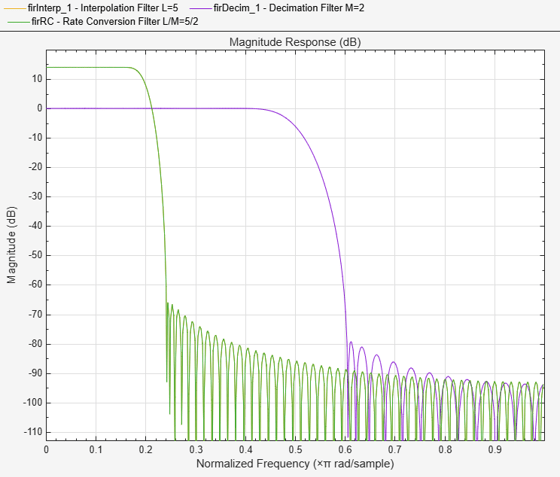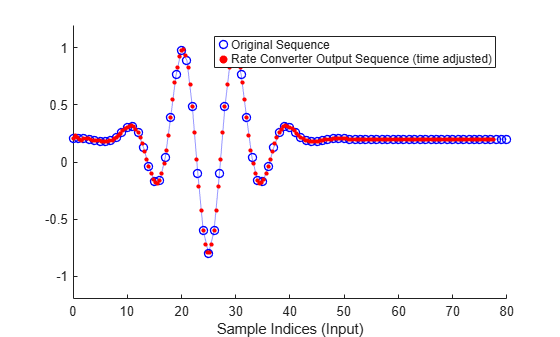# Design of Decimators/Interpolators

This example shows how to design filters for decimation and interpolation. Typically lowpass filters are used for decimation and for interpolation. When decimating, lowpass filters are used to reduce the bandwidth of a signal prior to reducing the sampling rate. This is done to minimize aliasing due to the reduction in the sampling rate. When interpolating, lowpass filters are used to remove spectral images from the low-rate signal. For general notes on lowpass filter design see the example on Designing Low Pass FIR Filters.

### Input signal

The samples of the input signal we will be using are drawn from standard normal distribution to have a flat spectrum.

```HSource = dsp.SignalSource('SamplesPerFrame', 500); HSource.Signal = randn(1e6,1); % Gaussian white noise signal ```

### Design of Decimators

When decimating, the bandwidth of a signal is reduced to an appropriate value so that minimal aliasing occurs when reducing the sampling rate. Suppose a signal that occupies the full Nyquist interval (i.e. has been critically sampled) has a sampling rate of 800 Hz. The signal's energy extends up to 400 Hz. If we'd like to reduce the sampling rate by a factor of 4 to 200 Hz, significant aliasing will occur unless the bandwidth of the signal is also reduced by a factor of 4. Ideally, a perfect lowpass filter with a cutoff at 100 Hz would be used. In practice, several things will occur: The signal's components between 0 and 100 Hz will be slightly distorted by the passband ripple of a non-ideal lowpass filter; there will be some aliasing due to the finite stopband attenuation of the filter; the filter will have a transition band which will distort the signal in such band. The amount of distortion introduced by each of these effects can be controlled by designing an appropriate filter. In general, to obtain a better filter, a higher filter order will be required.

Let's start by designing a simple lowpass decimator with a decimation factor of 4.

```M = 4; % Decimation factor Fp = 80; % Passband-edge frequency Fst = 100; % Stopband-edge frequency Ap = 0.1; % Passband peak-to-peak ripple Ast = 80; % Minimum stopband attenuation Fs = 800; % Sampling frequency HfdDecim = fdesign.decimator(M,'lowpass',Fp,Fst,Ap,Ast,Fs) ```
```HfdDecim = decimator with properties: MultirateType: 'Decimator' Response: 'Lowpass' DecimationFactor: 4 Specification: 'Fp,Fst,Ap,Ast' Description: {4x1 cell} NormalizedFrequency: 0 Fs: 800 Fs_in: 800 Fs_out: 200 Fpass: 80 Fstop: 100 Apass: 0.1000 Astop: 80 ```

The specifications for the filter determine that a transition band of 20 Hz is acceptable between 80 and 100 Hz and that the minimum attenuation for out of band components is 80 dB. Also that the maximum distortion for the components of interest is 0.05 dB (half the peak-to-peak passband ripple). An equiripple filter that meets these specs can be easily obtained as follows:

```HDecim = design(HfdDecim,'equiripple', 'SystemObject', true); measure(HDecim) HSpec = dsp.SpectrumAnalyzer(... % Spectrum scope 'PlotAsTwoSidedSpectrum', false, ... 'SpectralAverages', 50, 'OverlapPercent', 50, ... 'Title', 'Decimator with equiripple lowpass filter',... 'YLimits', [-50, 0], 'SampleRate', Fs/M*2); while ~isDone(HSource) inputSig = HSource(); % Input decimatedSig = HDecim(inputSig); % Decimator HSpec(upsample(decimatedSig,2)); % Spectrum % The upsampling is done to increase X-limits of SpectrumAnalyzer % beyond (1/M)*Fs/2 for better visualization end release(HSpec); reset(HSource); ```
```ans = Sample Rate : 800 Hz Passband Edge : 80 Hz 3-dB Point : 85.621 Hz 6-dB Point : 87.8492 Hz Stopband Edge : 100 Hz Passband Ripple : 0.092414 dB Stopband Atten. : 80.3135 dB Transition Width : 20 Hz ```It is clear from the measurements that the design meets the specs.

### Using Nyquist Filters

Nyquist filters are attractive for decimation and interpolation due to the fact that a 1/M fraction of the number of coefficients is zero. The band of the Nyquist filter is typically set to be equal to the decimation factor, this centers the cutoff frequency at (1/M)*Fs/2. In this example, the transition band is centered around (1/4)*400 = 100 Hz.

```TW = 20; % Transition width of 20 Hz HfdNyqDecim = fdesign.decimator(M,'nyquist',M,TW,Ast,Fs) ```
```HfdNyqDecim = decimator with properties: MultirateType: 'Decimator' Response: 'Nyquist' DecimationFactor: 4 Specification: 'TW,Ast' Description: {2x1 cell} Band: 4 NormalizedFrequency: 0 Fs: 800 Fs_in: 800 Fs_out: 200 TransitionWidth: 20 Astop: 80 ```

A Kaiser window design can be obtained in a straightforward manner.

```HNyqDecim = design(HfdNyqDecim,'kaiserwin','SystemObject', true); HSpec2 = dsp.SpectrumAnalyzer('PlotAsTwoSidedSpectrum', false, ... 'SpectralAverages', 50, 'OverlapPercent', 50, ... 'Title', 'Decimator with Nyquist filter',... 'YLimits', [-50, 0],... 'SampleRate', Fs/M*2); % Spectrum scope while ~isDone(HSource) inputSig = HSource(); % Input decimatedSig = HNyqDecim(inputSig); % Decimator HSpec2(upsample(decimatedSig,2)); % Spectrum % The upsampling is done to increase X-limits of SpectrumAnalyzer % beyond (1/M)*Fs/2 for better visualization end release(HSpec2); reset(HSource); ```### Aliasing with Nyquist Filters

Suppose the signal to be filtered has a flat spectrum. Once filtered, it acquires the spectral shape of the filter. After reducing the sampling rate, this spectrum is repeated with replicas centered around multiples of the new lower sampling frequency. An illustration of the spectrum of the decimated signal can be found from:

```NFFT = 4096; [H,f] = freqz(HNyqDecim,NFFT,'whole',Fs); figure; plot(f-Fs/2,20*log10(abs(fftshift(H)))) grid on hold on plot(f-Fs/M,20*log10(abs(fftshift(H))),'r-') plot(f-Fs/2-Fs/M,20*log10(abs(fftshift(H))),'k-') legend('Baseband spectrum', ... 'First positive replica', 'First negative replica') title('Aliasing with Nyquist filter'); fig = gcf; fig.Color = 'White'; hold off ```Note that the replicas overlap somewhat, so aliasing is introduced. However, the aliasing only occurs in the transition band. That is, significant energy (above the prescribed 80 dB) from the first replica only aliases into the baseband between 90 and 100 Hz. Since the filter was transitioning in this region anyway, the signal has been distorted in that band and aliasing there is not important.

On the other hand, notice that although we have used the same transition width as with the lowpass design from above, we actually retain a wider usable band (90 Hz rather than 80) when comparing this Nyquist design with the original lowpass design. To illustrate this, let's follow the same procedure to plot the spectrum of the decimated signal when the lowpass design from above is used

```[H,f] = freqz(HDecim,NFFT,'whole',Fs); figure; plot(f-Fs/2,20*log10(abs(fftshift(H)))) grid on hold on plot(f-Fs/M,20*log10(abs(fftshift(H))),'r-') plot(f-Fs/2-Fs/M,20*log10(abs(fftshift(H))),'k-') legend('Baseband spectrum', ... 'First positive replica', 'First negative replica') title('Aliasing with lowpass filter'); fig = gcf; fig.Color = 'White'; hold off ```In this case, there is no significant overlap (above 80 dB) between replicas, however because the transition region started at 80 Hz, the resulting decimated signal has a smaller usable bandwidth.

### Decimating by 2: Halfband Filters

When the decimation factor is 2, the Nyquist filter becomes a halfband filter. These filters are very attractive because just about half of their coefficients are equal to zero. Often, to design Nyquist filters when the band is an even number, it is desirable to perform a multistage design that uses halfband filters in some/all of the stages.

```HfdHBDecim = fdesign.decimator(2,'halfband'); HHBDecim = design(HfdHBDecim,'equiripple','SystemObject', true); HSpec3 = dsp.SpectrumAnalyzer('PlotAsTwoSidedSpectrum', false, ... 'SpectralAverages', 50, 'OverlapPercent', 50, ... 'Title', 'Decimator with halfband filter',... 'YLimits', [-50, 0],... 'SampleRate', Fs); % Spectrum scope while ~isDone(HSource) inputSig = HSource(); % Input decimatedSig = HHBDecim(inputSig); % Decimator HSpec3(upsample(decimatedSig,2)); % Spectrum end release(HSpec3); reset(HSource); ```As with other Nyquist filters, when halfbands are used for decimation, aliasing will occur only in the transition region.

### Interpolation

When interpolating a signal, the baseband response of the signal should be left as unaltered as possible. Interpolation is obtained by removing spectral replicas when the sampling rate is increased.

Suppose we have a signal sampled at 48 Hz. If it is critically sampled, there is significant energy in the signal up to 24 Hz. If we want to interpolate by a factor of 4, we would ideally design a lowpass filter running at 192 Hz with a cutoff at 24 Hz. As with decimation, in practice an acceptable transition width needs to be incorporated into the design of the lowpass filter used for interpolation along with passband ripple and a finite stopband attenuation. For example, consider the following specs:

```L = 4; % Interpolation factor Fp = 22; % Passband-edge frequency Fst = 24; % Stopband-edge frequency Ap = 0.1; % Passband peak-to-peak ripple Ast = 80; % Minimum stopband attenuation Fs = 48; % Sampling frequency HfdInterp = fdesign.interpolator(L,'lowpass',Fp,Fst,Ap,Ast,Fs*L) ```
```HfdInterp = interpolator with properties: MultirateType: 'Interpolator' Response: 'Lowpass' InterpolationFactor: 4 Specification: 'Fp,Fst,Ap,Ast' Description: {4x1 cell} NormalizedFrequency: 0 Fs: 192 Fs_in: 48 Fs_out: 192 Fpass: 22 Fstop: 24 Apass: 0.1000 Astop: 80 ```

An equiripple design that meets the specs can be found in the same manner as with decimators

```HInterp = design(HfdInterp,'equiripple','SystemObject', true); HSpec4 = dsp.SpectrumAnalyzer('PlotAsTwoSidedSpectrum', false, ... 'SpectralAverages', 50, 'OverlapPercent', 50, ... 'Title', 'Interpolator with equiripple lowpass filter',... 'SampleRate', Fs*L); % Spectrum scope while ~isDone(HSource) inputSig = HSource(); % Input interpSig = HInterp(inputSig); % Interpolator HSpec4(interpSig); % Spectrum end release(HSpec4); reset(HSource); ```Notice that the filter has a gain of 6 dBm. In general interpolators will have a gain equal to the interpolation factor. This is needed for the signal being interpolated to maintain the same range after interpolation. For example,

```release(HInterp); HSin = dsp.SineWave('Frequency', 18, 'SampleRate', Fs, ... 'SamplesPerFrame', 100); interpSig = HInterp(HSin()); HPlot = dsp.ArrayPlot('YLimits', [-2, 2], ... 'Title', 'Sine wave interpolated'); HPlot(interpSig(200:300)) % Plot the output ```Note that although the filter has a gain of 4, the interpolated signal has the same amplitude as the original signal.

### Use of Nyquist Filters for Interpolation

Similar to the decimation case, Nyquist filters are attractive for interpolation purposes. Moreover, given that there is a coefficient equal to zero every L samples, the use of Nyquist filters ensures that the samples from the input signal are retained unaltered at the output. This is not the case for other lowpass filters when used for interpolation (on the other hand, distortion may be minimal in other filters, so this is not necessarily a huge deal).

```TW = 2; HfdNyqInterp = fdesign.interpolator(L,'nyquist',L,TW,Ast,Fs*L) HNyqInterp = design(HfdNyqInterp,'kaiserwin', 'SystemObject', true); HSpec5 = dsp.SpectrumAnalyzer('PlotAsTwoSidedSpectrum', false, ... 'SpectralAverages', 30, 'OverlapPercent', 50, ... 'Title', 'Interpolator with Nyquist filter',... 'SampleRate', Fs*L); % Spectrum scope while ~isDone(HSource) inputSig = HSource(); % Input interpSig = HNyqInterp(inputSig); % Decimator HSpec5(interpSig); % Spectrum end release(HSpec5); reset(HSource); ```
```HfdNyqInterp = interpolator with properties: MultirateType: 'Interpolator' Response: 'Nyquist' InterpolationFactor: 4 Specification: 'TW,Ast' Description: {2x1 cell} Band: 4 NormalizedFrequency: 0 Fs: 192 Fs_in: 48 Fs_out: 192 TransitionWidth: 2 Astop: 80 ```In an analogous manner to decimation, when used for interpolation, Nyquist filters allow some degree of imaging. That is, some frequencies above the cutoff frequency are not attenuated by the value of Ast. However, this occurs only in the transition band of the filter. On the other hand, once again a wider portion of the baseband of the original signal is maintained intact when compared to a lowpass filter with stopband-edge at the ideal cutoff frequency when both filters have the same transition width.

Watch now